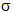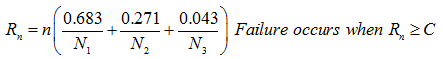you are here:/ News & Insights / Engineering Advantage Blog / How Do I Calculate Fatigue in a Random Vibration Environment? - Part 1

# How Do I Calculate Fatigue in a Random Vibration Environment? - Part 1December 4, 2015 By: Eric Stamper

In a related blog "How to Calculate Fatigue Life When the Load History is Complex", the ground work for calculating fatigue life is discussed. In this post, I'll build upon the discussion of fatigue failure and apply it to a Random Vibration load history.

In a random vibration analysis, it is assumed that the loading and response is statistical in nature and it can be represented by a zero-mean normal (Gaussian) distribution. It is sometimes convenient to view this distribution from the perspective of the likelihood that a certain level of load or response will fall within a certain standard deviation from the mean. Typically, we consider the 1, 2, and 3(standard deviation) levels. As an example, given a random Gaussian loading, x(t), the probability that x(t) lies between ± 1is 68.3% (e.g the majority of the loading over time is assumed to occur near the nominal levels with the peaks occurring less frequently), the probability that it lies between ± 2is 95.4%, and  that for ± 3is 99.7%, as shown in Figure 1 above.

Time-domain methods, using rainflow counting, as described in the aforementioned blog post, can also be applied to random processes. However, analysis in the frequency domain is usually preferred due to the significant advantage from the perspective of numerical computation. There are many frequency-based methods that have been developed over the years which calculate damage based on a random vibration loading. These different methods employ various techniques that calculate the fatigue life based on the 1values that are typically calculated by the Finite Element Analysis. For example, an FEA calculated maximum stress of Smax = 5ksi indicates a 68.3% probability (1) that Smax will be 5ksi or less. A method to calculate fatigue life, therefore needs to be selected and applied to these results to account for the statistical variability (unless a 68.3% of survivability is acceptable). One of the simplest approaches that can be used for design is to just assume that ± 3gives infinite life based on a Goodman diagram (i.e. a 99.7% probability (3) that Smax will be 3x5ksi = 15ksi or less). This approach can often be too conservative, in which case, more sophisticated methods are available.

All the common methods used today are based on the Palmgren-Miner hypothesis, which asserts that fatigue damage is cumulative, proportional to the applied levels of applied stress, and that the damage is independent of the order in which the stresses are applied.

Using this technique, structural performance is evaluated by comparing the calculated cumulative damage ratio to a specified cumulative damage index.

The Steinberg 3-band method for damage calculation is frequently used due to its simplicity (Ref 1). It uses a Miner's Rule approach to calculate cumulative fatigue damage by assuming that the stress amplitude response at a given location has a Gaussian distribution that's divided into the following three intervals:

• 68.3% of the time at 1• 27.1% of the time at 2• 4.3% of the time at 3In each of these intervals, the number of cycles to failure (N1, N2, and N3) can be determined from the material S-n curve. Then, if the total number of applied cycles "n" is known, we can use the Steinberg 3-band method as shown in Figure 2 to determine the cumulative fatigue damage index, Rn.When all the life is used up, the value of Rn will be equal to 1. However, due to the uncertainties of this method, safety factors are built in via the failure index, C. The value of C to be used is dependent on the industry and application. For example, a value of 1 can be too high based on test data for electronics, and might be lowered to 0.7 or 0.3.

Stay tuned for Part 2 of this post, where I'll walk through a sample calculation using this method. I'll also discuss other methods, which can produce better correlation to results for a random response. If you have any experience with calculating fatigue damage, please leave a comment! I'd like to hear about your approach.